# RD Sharma Solutions - Chapter 18 - Practical Geometry (Constructions) (Part - 5), Class 8, Maths Notes | Study RD Sharma Solutions for Class 8 Mathematics - Class 8

## Class 8: RD Sharma Solutions - Chapter 18 - Practical Geometry (Constructions) (Part - 5), Class 8, Maths Notes | Study RD Sharma Solutions for Class 8 Mathematics - Class 8

The document RD Sharma Solutions - Chapter 18 - Practical Geometry (Constructions) (Part - 5), Class 8, Maths Notes | Study RD Sharma Solutions for Class 8 Mathematics - Class 8 is a part of the Class 8 Course RD Sharma Solutions for Class 8 Mathematics.
All you need of Class 8 at this link: Class 8

PAGE NO 18.8:

Question 1:

Construct a quadrilateral ABCD in which AB  = 3.8 cm, BC  = 3.4 cm, CD  = 4.5 cm, AD  = 5 cm and ∠B  = 80°.

Steps of construction:

Step I: Draw AB = 3.8 cm.

Step II: Construct ∠ABC = 80°.

Step III : With B as the centre and radius 3.4 cm, cut off BC = 3.4 cm.

Step IV: With C as the centre and radius 4.5 cm, draw an arc.

Step V :With A as the centre and radius 5.3 cm, draw an arc to intersect the arc drawn in Step IV at D.

Step VI: Join AD, BC and CD to obtained the required quadrilateral.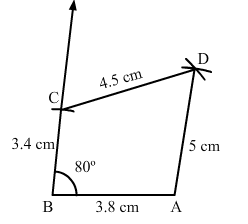Question 2:

Construct a quadrilateral ABCD, given that AB  = 8 cm, BC  = 8 cm, CD  = 10 cm, AD  = 10 cm and ∠A  = 45°.

Steps of Construction:

Step I: Draw AB = 8 cm.

Step II: Construct ∠BAD = 45°.

Step III : With A as the centre and radius 10 cm, cut off AD = 10 cm.

Step IV: With D as the centre and radius 10 cm, draw an arc.

Step V :With B as the centre and radius 8 cm, draw an arc to intersect the arc drawn in Step IV at C.

Step VI: Join  BC and CD to obtained the required quadrilateral.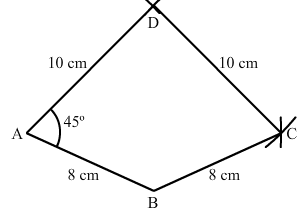Question 3:

Construct a quadrilateral ABCD in which AB  = 7.7 cm, BC  = 6.8 cm, CD  = 5.1 cm, AD  = 3.6 cm and ∠C  = 120°.

Steps of construction:

Step I: Draw DC = 5.1 cm.

Step II: Construct ∠DCB = 120°.

Step III : With C as the centre and radius 6.8 cm, cut off BC = 6.8 cm.

Step IV: With B as the centre and radius 7.7 cm, draw an arc.

Step V :With D as the centre and radius 3.6 cm, draw an arc to intersect the arc drawn in Step IV at A.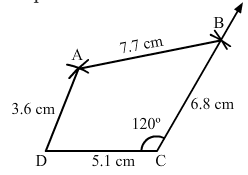Question 4:

Construct a quadrilateral ABCD in which AB  =  BC  = 3 cm, AD  =  CD  = 5 cm, and ∠B  = 120°.

Steps of construction:

Step I: Draw AB = 3 cm.

Step II: Construct ∠ABC = 120°.

Step III : With B as the centre and radius 3 cm, cut off BC = 3 cm.

Step IV: With C as the centre and radius 5 cm, draw an arc.

Step V :With A as the centre and radius 5 cm, draw an arc to intersect the arc drawn in Step IV at D.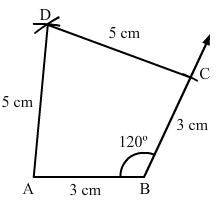Question 5:

Construct a quadrilateral ABCD in which AB  = 2.8 cm, BC  = 3.1 cm, CD  = 2.6 cm, and DA  = 3.3 cm and ∠A  = 60°.

Steps of construction:

Step I: Draw AB = 2.8 cm.

Step II: Construct ∠BAD = 60°.

Step III : With A as the centre and radius 3.3 cm, cut off AD = 3.3 cm.

Step IV: With D as the centre and radius 2.6 cm, draw an arc.

Step V :With B as the centre and radius 3.1 cm, draw an arc to intersect the arc drawn in Step IV at C.

Step VI: Join BC and CD to obtained the required quadrilateral.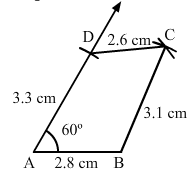Question 6:

Construct a quadrilateral ABCD in which AB  =  BC  = 6 cm, AD  =  DC  = 4.5 cm and ∠B  = 120°.

Steps of construction:

Step I: Draw AB = 6 cm.

Step II: Construct ∠ABC = 120°.

Step III : With B as the centre and radius 6 cm, cut off BC = 6 cm.

Now, we can see that AC is about 10.3 cm

which is greater than AD+CD = 4.5+4.5 = 9 cm.

We know that sum of the lengths of two sides of triangle is always greater than the third side but here, the sum of AD and CD is less than AC.

So, construction of the given quadrilateral is not possible.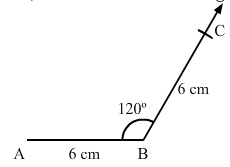The document RD Sharma Solutions - Chapter 18 - Practical Geometry (Constructions) (Part - 5), Class 8, Maths Notes | Study RD Sharma Solutions for Class 8 Mathematics - Class 8 is a part of the Class 8 Course RD Sharma Solutions for Class 8 Mathematics.
All you need of Class 8 at this link: Class 8Use Code STAYHOME200 and get INR 200 additional OFF

## RD Sharma Solutions for Class 8 Mathematics

88 docs

Track your progress, build streaks, highlight & save important lessons and more!

,

,

,

,

,

,

,

,

,

,

,

,

,

,

,

,

,

,

,

,

,

,

,

,

,

,

,

;